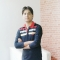## bin() in PythonUpdated on 07-Aug-2019 07:57:15

The bin() function converts a decimal to binary. You can use a positive or negative integer as the parameter to be converted.SyntaxBelow is the syntax of the function.bin(n) Parameters : an integer to convert Return Value : A binary string of an integer or int object. Exceptions : Raises TypeError ... Read More

## Backward iteration in PythonUpdated on 07-Aug-2019 07:52:06

Sometimes we need to go through the elements of a list in backward order. To achieve this we need to read the last element first and then the last but one and so on till the element at index 0. Various python programming features can be used to achieve this.Using ... Read More

## Ascii() in pythonUpdated on 07-Aug-2019 07:50:08

ASCII is abbreviated from of American Standard Code for Information Interchange. It is a character encoding standard. For example the ASCII value of English alphabet A is 65. Python provides this function to get the printable representation of an object. It takes one parameter which is an object that can ... Read More

## Apply uppercase to a column in Pandas dataframeUpdated on 07-Aug-2019 07:47:54

Exampleimport pandas as pd # A dataframe df = pd.DataFrame({'Day': ['mon', 'tue', 'wed', 'thu', 'fri'], 'Subject': ['Math', 'english', 'science', 'music', 'games']}) df['Day'] = df['Day'].str.upper() print(df)OutputRunning the above code gives us the following result −     Day       Subject 0    MON       Math 1    TUE ... Read More

## Apply function to every row in a Pandas DataFrameUpdated on 07-Aug-2019 07:44:34

By applying a lambda function to each row Exampleimport pandas as pd df = pd.DataFrame([(10, 3, 13), (0, 42, 11), (26, 52, 1)], columns=list('xyz')) print("Existing matrix") print(df) NewMatrix = df.apply(lambda a: a + 10, axis=1) print("Modified Matrix") print(NewMatrix)OutputRunning the above code gives us the following result −Existing matrix      x ... Read More

## append() and extend() in PythonUpdated on 07-Aug-2019 07:39:41

The append() and extend() functions are used with the python list to increase its number of elements. But the two have different behaviors as shown below.append()Syntax: list_name.append(‘value’) It takes only one argument.This function appends the incoming element to the end of the list as a single new element. Even if ... Read More

## Add a row at top in pandas DataFrameUpdated on 07-Aug-2019 07:33:33

In Pandas a DataFrame is a two-dimensional data structure, i.e., data is aligned in a tabular fashion in rows and columns. We can create a DataFrame using list, dict, series and another DataFrame. But when we want to add a new row to an already created DataFrame, it is achieved ... Read More

## Absolute and Relative Imports in Python